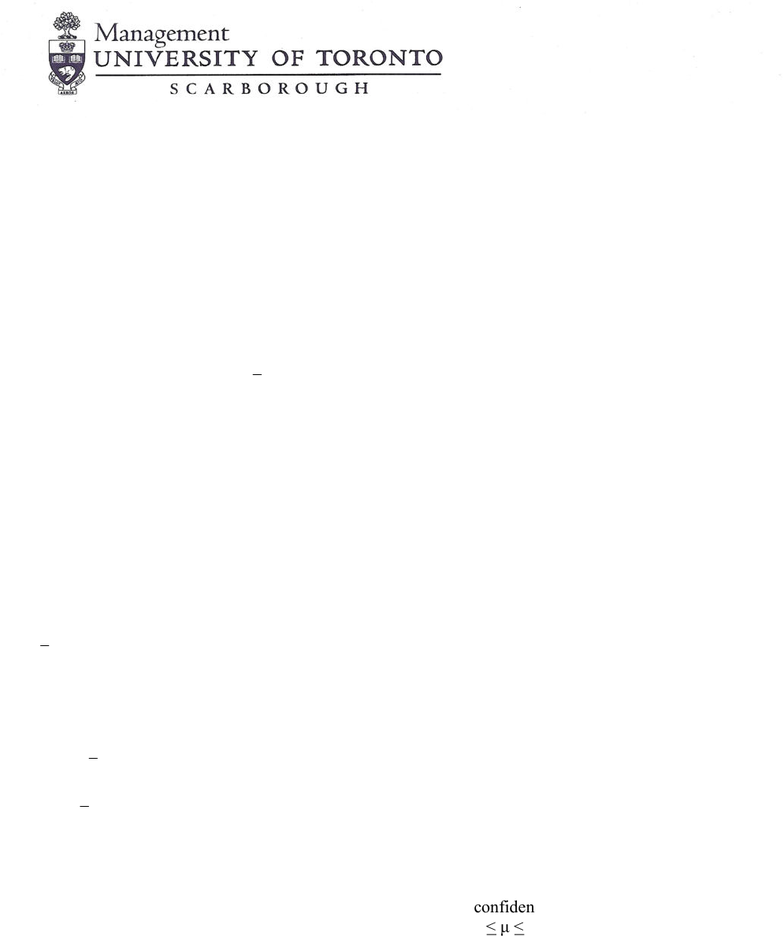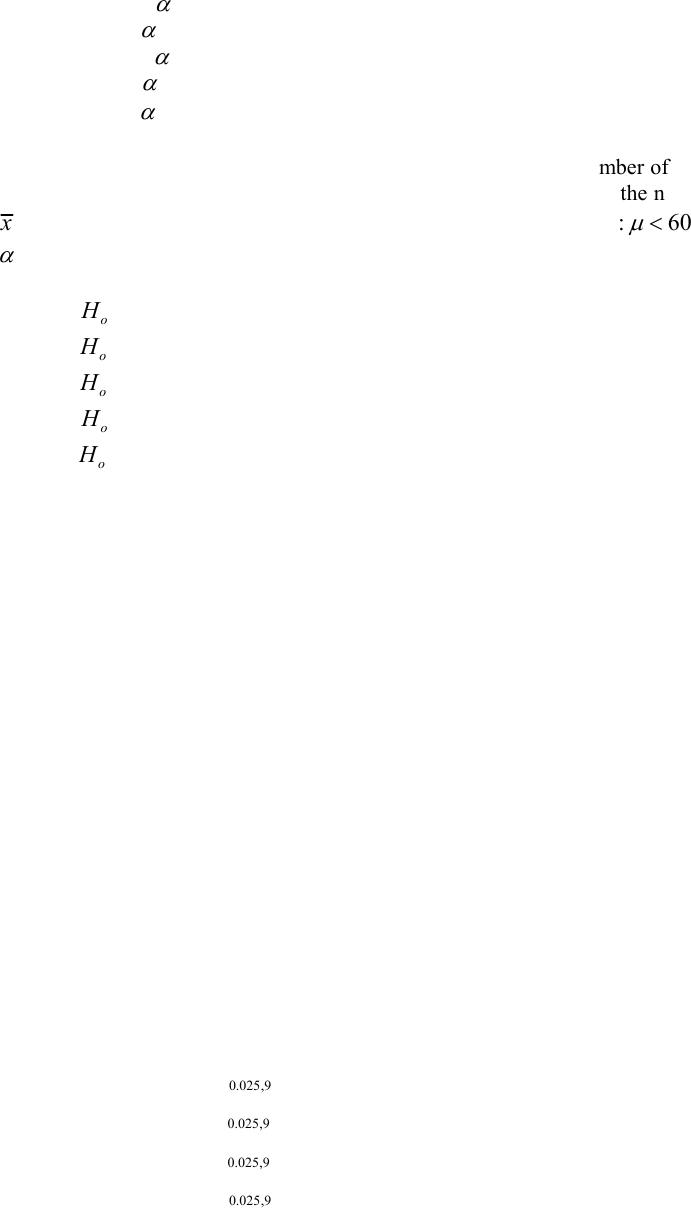# MGEB12H3 Lecture Notes - Lecture 1: Simple Random Sample, Null Hypothesis, Interval Estimation

86 views10 pages
School
UTSC
Department
Economics for Management Studies
Course
MGEB12H3
ProfessorMGEB12: Quantitative Methods in Economics-II
Problem Set-1
Chpater-9: 4a, 6, 10, 12, 14, 20, 22, 24, 26, 30, 34
Supplemental:
1. Suppose a random sample of size 25 is selected from a population with mean μ, the value of which is
unknown. The sample statistics are 4.6x, s = 10. In the test:
H0: μ = 10
H1: μ < 10 using α = .05.
Which of the following statements do you KNOW is correct?
A. A type 1 error has been committed.
B. H0 is rejected.
C. H0 is not rejected.
D. Statements (A) and (B) are correct.
E. None of the above.
2. If one does not reject the null hypothesis Ho:
= 20, and thus does not accept the alternative H1:
< 20,
with x=10, then
A. a mistake has been made because the null hypothesis should be Ho:
20, if the alternative hypothesis is
Ha:
< 20.
B. at the same significance level, the null hypothesis Ho:
= 0 can not be rejected in favor of the alternative Ha:
> 10, for x=10.
C. at the same significance level, the null hypothesis Ho:
= 0 can not be rejected in favor of the alternative Ha:
> 0, for x=10.
D. there is statistical proof that
is 20 and that it is not less than or greater than 20.
E. there is statistical proof that
is 20 and that it is not less than 20.
3. An analyst, using a simple random sample, obtained a 99 percent confidence interval for mean monthly
family income in a Toronto suburb with the following results: \$3,200 < μ < \$10,000. If the analyst had used a 90
percent confidence instead of a 99 percent one, then the interval would be:
A. shorter and would involve a larger risk of being an incorrect interval estimate.
B. longer and would involve a smaller risk of being an incorrect interval estimate.
C. shorter and would involve a smaller risk of being an incorrect interval estimate.
D. longer and would involve a larger risk of being an incorrect interval estimate.
E. cannot say without knowing the sample mean
Unlock document

This preview shows pages 1-3 of the document.
Unlock all 10 pages and 3 million more documents.2
4. The p-value of a test:
A. is the smallest
at which the null hypothesis can be rejected
B. is the largest
at which the null hypothesis can be rejected
C. is the smallest
at which the null hypothesis cannot be rejected
D. is the largest
at which the null hypothesis cannot be rejected
E. increases if
increases
5. Researchers determined that 60 Kleenex tissues is the average number of tissues used during a cold. Suppose
a random sample of 100 Kleenex users yielded the following data on the number of tissues used during a cold:
x
= 52 and s = 22. Suppose the alternative we wanted to test was 1
: 60
H
. The correct rejection region for
= 0.05 is
A. reject
o
if t > 1.6604
B. reject
o
if t < - 1.6604
C. reject
o
if t > 1.9842 or Z < - 1.9842
D. reject
o
if t < - 1.9842
E. reject
o
if t > 1.9842
6. A random sample of size 15 taken from a normally distributed population revealed a sample mean of 75 and a
sample variance of 25. The upper limit of a 95% confidence interval for the population mean would equal:
A. 72.231
B. 72.727
C. 77.273
D. 73.241
E. 77.769
Questions 7-8: A random sample of 10 college students was drawn from a large university with normal
distribution. Their ages have an average of 21.3 and standard deviation equal to 3.20.
7. The 90% confidence for the age of the population is.
A. LCL = 19.791, and UCL = 22.809
B. LCL = 19.446, and UCL = 23.154
C. LCL = 18.888, and UCL = 23.712
D. LCL = 18.467, and UCL = 24.133
E. LCL = 19.067, and UCL = 23.554
8. Test to determine if we can infer at the 5% significance level that the population mean is not equal to 20:
A. Rejection region: |t| >
0.025,9
t= 2.262, Test statistic: t = 1.285
B. Rejection region: |t| >
0.025,9
t= 1.833, Test statistic: t = 1.285
C. Rejection region: |t| >
0.025,9
t= 2.262, Test statistic: t = 1.241
D. Rejection region: |t| >
0.025,9
t= 1.833, Test statistic: t = 1.241
E. The sample size is too small cannot use the table.
Unlock document

This preview shows pages 1-3 of the document.
Unlock all 10 pages and 3 million more documents.

3
9. In testing the hypothesis 100:
0
H vs. 100:
1
H, the p-value is found to be 0.074, and the sample
mean is 105. Which of the following statements is true?
A. The probability of observing a sample mean at least as large as 105 from a population whose mean is 100 is
0.074.
B. The probability of observing a sample mean smaller than 105 from a population whose mean is 100 is 0.074
C. The probability that the population mean is larger than 100 is 0.074
D. The probability that the margin of error is larger than 5 is 0.074
E. None of the above
10. In a given hypothesis test, the null hypothesis can be rejected at the .10 and .05 level of significance, but
cannot be rejected at the .01 level. The most accurate statement that can be made about the p-value for this test
is that:
A. p-value = 0.01
B. p-value = 0.10
C. 0.01 < p-value < 0.05
D. 0.05 < p-value < 0.10
E. p-value < 0.01
11. The null and alternative hypotheses are stated as Ho: µ=10 and Ha: µ < 10. When a random sample of size n
= 36 is drawn, it yields a mean of 13; thus:
A. The null hypothesis can be rejected if the sample standard deviation is 6 and the probability of a type I error
is relatively large at 0.10, but not for a small alpha = 0.01.
B. The null hypothesis can be rejected for any of the typical type I error levels (0.10, 0.05, or 0.01) because the
p-value is smaller.
C. The null hypothesis cannot be rejected regardless of the type I error level.
D. The alternative hypothesis can be accepted at any of the type I error levels.
E. None of the above
12. The prices of CD players are normally distributed, with a standard deviation of \$12. A random sample of 16
CD player prices is drawn. A confidence interval for the mean price shows a \$9.30 margin of error. What was
the level of confidence?
A. 99.9%
B. 99.8%
C. 99.7%
D. 99.6%
E. 99.5%
13. One characteristic of any Student’s t distribution is
A. it is right skewed.
B. it is left skewed.
C. as n increases, the t-distribution approaches a uniform distribution.
D. it is described by its degrees of freedom.
E. it has a mean of 0 and a standard deviation of 1.
14. The 95% confidence interval for μ is between [10, 15]. What conclusion can you make if you test H0: μ = 16
versus H1: μ # 16 at α = 0.05?
A. Reject the null and conclude the alternative.
B. Fail to reject the null.
Unlock document

This preview shows pages 1-3 of the document.
Unlock all 10 pages and 3 million more documents.